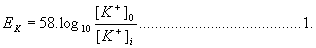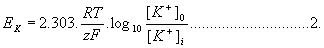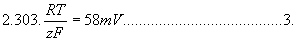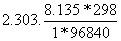# The Nernst equation

You probably recognise equation 1 as the Nernst equation.This equation defines the relation between the concentrations of an ion on either side of a membrane that it perfectly selective for that ion and the potential difference (voltage) that will be measured across that membrane under equilibrium conditions.

As we discussed in the practical class, the Nernst equation is able to give a value for the voltage that must exist across the membrane in order to balance a chemical gradient that exists for the ion in question.

So, you should know (from your lectures that) a plot of log of the K concentration (x axis) against membrane potential gives a straight line (is a linear function) if the membrane is perfectly selective for potassium ions. You also learned that the slope of the line should have a value of 58 mV for a 10-fold concentration difference at room temperature (rising to about 61 at 37o C). This is demonstrated in figure 1

Table 1

 [K]bath (mM) 1 3 10 30 100 300 EK (mV) -124.5 -96.8 -66.5 -38.8 -8.5 +19.2 Figure 1.The arrows in figure 1 are separated by 1 log unit, or put another way, they mark a 10-fold concentration difference. The membrane potential difference between these two points is 58 mV (difference between -66.5 and -8.5 mV).

You may have wondered why it should be 58 mV? What is so magical about the number 58??? Perhaps you have been puzzled by different values in textbooks, 61 mV, perhaps. The explanation is that equation 1 is rather simplified. Some of you may already have seen that the Nernst equation is expressed in another form that is used in some textbooks:Where:

2.303 is a conversion factor from natural log to log10

R = Gas constant, 8.135 J K-1 mol-1

T = temperature in K (273 + temp in oC)

z = valency of ion (Na+ is plus one, Ca2+ is plus two and Cl- is minus one)

F = Faraday’s constant, 9.684 x 104 C mol-1

So it follows that at room temperature:So the value 58 mV comes not out of thin air but from the terms in equation 3. Please don't panic. If you remember the Nernst potential as equation 1, you'll be fine, but you should remember that the number is 61 mV (and not 58 mV) at mammalian body temperature. Nevertheless, there are textbooks that give the Nernst equation as shown in equation 2.

Because of this I think it’s a good idea to look at equations 1& 2 and find out why the answer to Life, the Universe and Everything is 58 mV!

## Conditions and Assumptions

Since the Nernst equation is concerned with the movement of a single ion species through selective channels, you need be concerned only with the concentration of that ion. So when dealing with K+, as above, you need not worry about Na+ (there is another equation that takes other ions into account - do you know what it is called?)

Let's first show that 2.303*RT/zF has the value 58 mV at room temperature.

R = 8.135; T = 273 + 25 (room temperature of 25 oC); z = 1; F = 9.684 x 104= 0.0578

Accept for now that this has the units of Volts. Since this is a small value it is most conveniently given in mV (1/1000 of a volt), in which case it becomes 57.8 mV. For most purposes this is rounded up to 58 mV.

OK, what about the units. Why should RT/zF have the units, 'volts'?

## The effect of valency (the charge on the ion)

Look again at the equation RT/zF. The examples that we've been looking at are for ions like Na+ and K+ which have a charge of +1 so the term z is +1. What about Ca2+ which has a charge of 2+? This will double the value of zF. What effect will this have. Specifically, what should the Nernst slope be for a membrane that is selective for Ca2+ ions? Click here when you have decided.